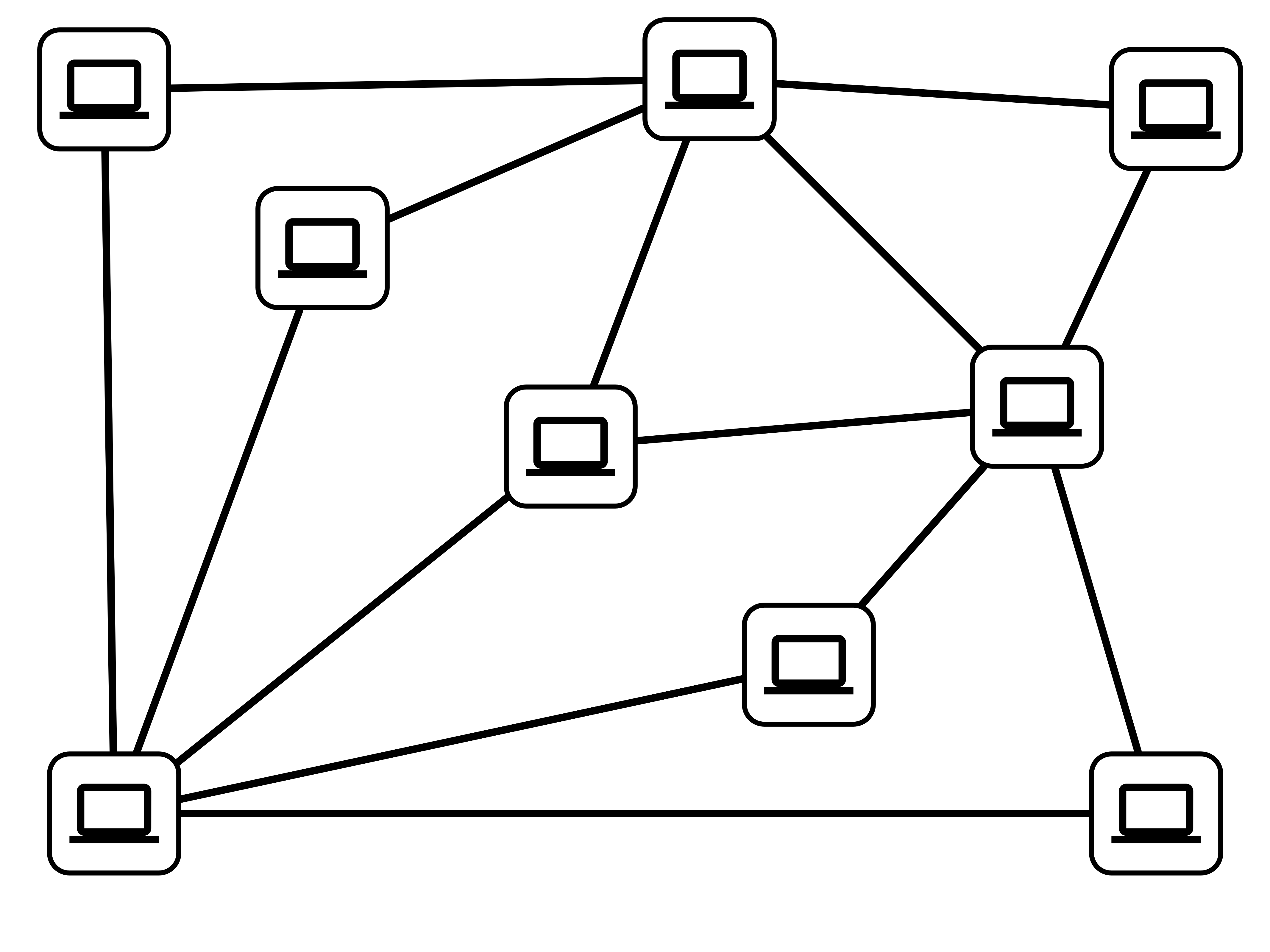# Lecture 34: P and NP

$\def\verify{ {\mathrm{verify}} }$

## Announcement

Job Candidate Talk TODAY

Sims Osborne, UNC Chapel Hill

Using Simultaneous Multithreading to Support Real-Time Scheduling

• 4:00 in SCCE A131
• Refreshments at 3:30 in SCCE C209

## Homework 6

Posted soon…

…not to be turned in!

• solution posted later this week

## Last Time

Two Problems:

• Minimum Vertex Cover (MVC)
• Maximum Indpendent Set (MaxIS)

Polynomial-time reductions between them:

• MVC $\leq_P$ MaxIS
• MaxIS $\leq_P$ MVC

Consequence

• MVC can be solved efficiently $\iff$ MaxIS can be solved efficiently

## Today

1. Decision Problems
2. The Classes P and NP

## A Technicality

Objective. Understand relationships between computational problems.

Technical issue. Desired outputs for different problems can be vastly different:

• matching
• independent set
• spanning tree

Convenience. Focus on decision problems:

• output is “yes”/”no”

## MVC vs VC

Minimum Vertex Cover (MVC)

• Input: Graph $G$

• Output: A vertex cover $C$ of smallest possible size

Vertex Cover (VC)

Input: Graph $G$, number $k$

Output:

• “yes” if $G$ has a vertex cover of size $k$
• “no” otherwise

## MaxIS vs IS

Maximum Independent Set (MaxIS)

Input: Graph $G$

Output: an indpendent set of the largest possible size

Independent Set (IS)

Input: Graph $G$, number $k$

Output:

• “yes” if $G$ has an indpendent set of size $k$
• “no” otherwise

## Complexity of Decision Problems

Goal. Classify (decision) problems according to their relative complexities:

• which problems can be solved efficiently?
• which problems cannot be solved efficiently?
• which problems can be reduced to other problems?

## The Class P

Definition. The class P consists of all decision problems that can be solved in polynomial time.

• P = “polynomial time”

• a problem $A$ is in P if there is an algorithm that given any instance $X$ of $A$

• the algorithm correctly outputs “yes”/”no”
• the running time is $O(N^c)$ for some constant $c$, $N$ = size of input

# The Class NP

## Verifying Output

Consider IS$(G, k)$:

• “yes” if $G$ has an indpendent set of size $k$
• “no” if $G$ does not have an indpendent set of size $k$Question. How could you be convinced that IS$(G, k)$ = “yes?”

## NP, Informally

NP = “nondeterministic polynomial time”

Informal Definition. The class NP consists of decision problems whose solution can be verified in polynomial time.

Setup

• $A$ is a decision problem, $X$ an instance (input) of $A$
• If $X$ is a “yes” instance, there should be some way to convince me this is the case
• If $X$ is a “no” instance, there should be no way to convince me $X$ is a “yes” instance

## Verifier

Definition. Given a decision problem A, a verifier for A is a polynomial time algorithm $\verify(X, C)$ that takes as input

• an instance $X$ of A, and
• a certificate $C$ (size polynomial in size of $X$)

and returns a value “accept” or “reject,” subject to two conditions:

1. completeness if $X$ is a “yes” instance, then there exists a certificate $C$ such that $\verify(X, C)$ returns “accept”

2. soundness if $X$ is a “no” instance, then for every certificate $C$, $\verify(X, C)$ returns “reject”

## A Verifier for IS

Consider IS$(G, k)$:

• “yes” if $G$ has an indpendent set of size $k$
• “no” if $G$ does not have an indpendent set of size $k$

What is a verifier for IS?

• what should be the certificate?
• how do we verify a certificate?

## NP, Formally

Definition. The class NP consists of all decision problems that admit a polynomial time verifier.

• By previous example, IS is in NP

Conceptually NP can be thought of the class of puzzles

• a puzzle may be hard to solve
• you can easily verify if you (or someone else) solved the puzzle

## P vs NP

Open Question. Is there any problem in NP that is not in P?

Informal statement. Are there problems that are hard to solve, but whose solutions are easy to verify?

• one of deepest mathematical challenges of our time

## Activity

Which of the following problems are in NP:

1. BipartiteMatching$(G, k)$
2. NoFlow$(G, k)$
3. GeneralizedChess$(n, C)$
4. BooleanSatisfiability$(\varphi(x_1, x_2, \ldots,x_n))$

## Bipartite Matching

Question. Is BipartiteMatching in NP?

## More Generally

If a problem $A$ is in P, then $A$ is in NP:

• P $\subseteq$ NP

Why?

## NoFlow

Question. Is NoFlow in NP?

## Observation

Even if did not know about the Ford-Fulkerson MaxFlow algorithm, we could still identify NoFlow is in NP.

How?

## GeneralizedChess

Question. Is GeneralizedChess in NP?

## Chess Remarks

A feature of chess games:

• a game may last exponentially many rounds in the size of the board
• therefore: winning strategy might require exponential time to describe/verify

Fact. GeneralizedChess requires exponential time to solve (in $n$).

Consequence. Showing GeneralizedChess is in NP would imply that P $\neq$ NP.

## Boolean Satisfiability

Question. Is BooleanSatisfiability in NP?

## Next Time

• NP Completeness: characterizing the “hardest” problems in NP